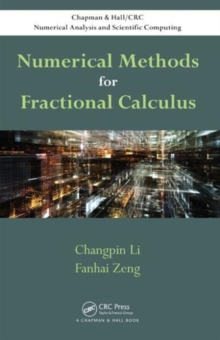Supporting your high street Find out how »
• My Account# Numerical Methods for Fractional Calculus Hardback

## Part of the Chapman & Hall/CRC Numerical Analysis and Scientific Computing Series series

#### Description

Numerical Methods for Fractional Calculus presents numerical methods for fractional integrals and fractional derivatives, finite difference methods for fractional ordinary differential equations (FODEs) and fractional partial differential equations (FPDEs), and finite element methods for FPDEs. The book introduces the basic definitions and properties of fractional integrals and derivatives before covering numerical methods for fractional integrals and derivatives.

It then discusses finite difference methods for both FODEs and FPDEs, including the Euler and linear multistep methods.

The final chapter shows how to solve FPDEs by using the finite element method. This book provides efficient and reliable numerical methods for solving fractional calculus problems.

It offers a primer for readers to further develop cutting-edge research in numerical fractional calculus.

MATLAB (R) functions are available on the book's CRC Press web page.

#### Information

• Format: Hardback
• Pages: 300 pages, 48 Tables, black and white; 12 Illustrations, black and white
• Publisher: Apple Academic Press Inc.
• Publication Date:
• Category: Calculus
• ISBN: 9781482253801

£95.00

£82.75£83.33

£75.00

£83.33

£75.00

£100.00

£92.85

£18.32

£16.49